# The SURVEYFREQ Procedure

#### Design Effect

If you specify the DEFF option in the TABLES statement, PROC SURVEYFREQ computes design effects for the overall proportion estimates in the frequency and crosstabulation tables. If you specify the ROW(DEFF) or COLUMN(DEFF) option, the procedure provides design effects for the row or column proportion estimates, respectively. The design effect for an estimate is the ratio of the actual variance (estimated based on the sample design) to the variance of a simple random sample with the same number of observations. See Lohr (2010) and Kish (1965) for details.

For Taylor series variance estimation, PROC SURVEYFREQ computes the design effect for the proportion in table cell (r, c) as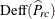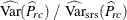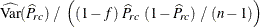where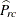is the estimate of the proportion in table cell (r, c),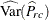is the variance of the estimate, f is the overall sampling fraction, and n is the sample size (unweighted frequency) for the two-way table.

For Taylor series variance estimation, PROC SURVEYFREQ determines the value of f, the overall sampling fraction, based on the RATE= or TOTAL= option. If you do not specify either of these options, PROC SURVEYFREQ assumes the value of f is negligible and does not use a finite population correction in the analysis, as described in the section Population Totals and Sampling Rates. If you specify RATE=value, PROC SURVEYFREQ uses this value as the overall sampling fraction f. If you specify TOTAL=value, PROC SURVEYFREQ computes f as the ratio of the number of PSUs in the sample to the specified total.

If you specify stratum sampling rates with the RATE=SAS-data-set option, then PROC SURVEYFREQ computes stratum totals based on these stratum sampling rates and the number of sample PSUs in each stratum. The procedure sums the stratum totals to form the overall total, and computes f as the ratio of the number of sample PSUs to the overall total. Alternatively, if you specify stratum totals with the TOTAL=SAS-data-set option, then PROC SURVEYFREQ sums these totals to compute the overall total. The overall sampling fraction f is then computed as the ratio of the number of sample PSUs to the overall total.

For BRR and jackknife variance estimation, PROC SURVEYFREQ computes the design effect for the proportion in table cell (r, c) as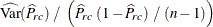whereis the estimate of the proportion in table cell (r, c),is the variance of the estimate, and n is the sample size (unweighted frequency) for the two-way table. This computation does not include the overall sampling fraction.

The procedure computes design effects similarly for proportions in one-way frequency tables, and also for row and column proportions in two-way tables. In these design effect computations, the value of n is the sample size (unweighted frequency) that corresponds to the total domain of the proportion estimate. For table cell proportions of a two-way table, the domain is the two-way table and the sample size n is the frequency of the two-way table. For row proportions, which are based on a two-way table row, the domain is the row and the sample size n is the frequency of the row.